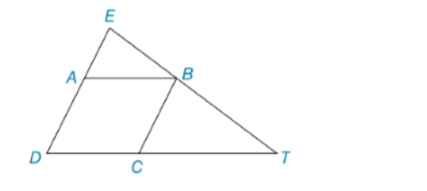Chapter 5.3, Problem 14EElementary Geometry For College St...

7th Edition
Alexander + 2 others
ISBN: 9781337614085

Solutions

Chapter
SectionElementary Geometry For College St...

7th Edition
Alexander + 2 others
ISBN: 9781337614085
Textbook Problem

In Exercises 13 to 16, provide the missing reasons.Given: Δ D E T  and  ▱ A B C D Prove: Δ A B E ∼ Δ C T BPROOF Statements Reasons 1. Δ D E T  and  ▱ A B C D 1. ? 2. A B ¯ ∥ D T ¯ 2. Opposite sides of a ▱ are ∥ 3. ∠ E B A ≅ ∠ T 3. ? 4. E D ¯ ∥ C B ¯ 4. ? 5. ∠ E ≅ ∠ C B T 5.? 5. Δ A B E ∼ Δ C T B 6. ?

To determine

To provide:

The missing reasons.

Explanation

Given:

Given: ΔDET and ABCD

Prove: ΔABEΔCTB

 PROOF Statements Reasons 1. ΔDET and ▱ABCD 1. ? 2. AB¯∥DT¯ 2. Opposite sides of ▱ are ∥ 3. ∠EBA≅∠T 3. ? 4. ED¯∥CB¯ 4. ? 5. ∠E≅∠CBT6. ΔABE∼ΔCTB 5. ?6. ?

Definition:

AA:

If the two angles of one triangle are congruent to the two angles of another triangle, then the triangles are similar.

Description:

Given that ΔDET and ABCD.

To Prove: ΔABEΔCTB

Given AB¯DT¯.

It is known that if two parallel lines AB and DT are cut by a transversal, corresponding angles are congruent.

Therefore, EBAT.

Since opposite sides of a are parallel, ED¯CB¯

Still sussing out bartleby?

Check out a sample textbook solution.

See a sample solution

The Solution to Your Study Problems

Bartleby provides explanations to thousands of textbook problems written by our experts, many with advanced degrees!

Get Started